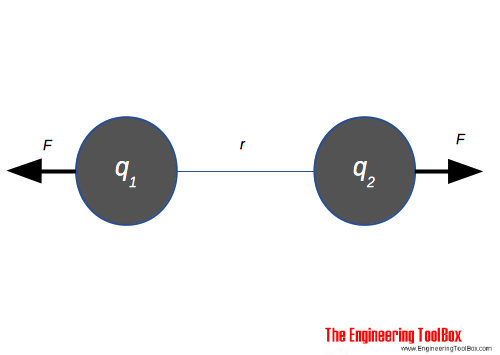Engineering ToolBox - Resources, Tools and Basic Information for Engineering and Design of Technical Applications!

# Coulomb's Law

## The electric force acting on a point charge.Coulomb's law calculates the electric force acting between to electric charges  q1 and q2 and can be expressed as

F = k q1 q2 / r2

= q1 q2 / (4 π ε0 r2)    (1)

where

F = force of attraction or repulsion (N)

k = Coulomb's constant = 1 / (4 π ε0) = 8.9875517873681764 × 109 (Nm2/C2) in air

q = charge (Coulombs, C)

r = distance between charges (m)

The quantity of charge (number of electrons) is measured in the unit Coulomb - C - where

1 coulomb (C) = 6.24 1018 electrons

The smallest charge that exists is the charge carried by an electron equal to -1.602 10-19 coulomb.

### Example - Force between two Charged Bodies

Two spheres in air with distance 20 mm is both charged with 2 μC (2 10-6 C). The force acting between the two spheres can be calculated as

F = (8.98755 109 Nm2/C2) ((2 μC) (10-6 C/μC)) ((2 μC) (10-6 C/μC)) / ((20 mm)(10-3 m/mm))2

= 90 N

## Related Topics

• ### Electrical

Electrical units, amps and electrical wiring, wire gauge and AWG, electrical formulas and motors.

## Related Documents

• ### Relative Permittivity - the Dielectric Constant

Common materials and their relative permittivity.

## Engineering ToolBox - SketchUp Extension - Online 3D modeling!

Add standard and customized parametric components - like flange beams, lumbers, piping, stairs and more - to your Sketchup model with the Engineering ToolBox - SketchUp Extension - enabled for use with older versions of the amazing SketchUp Make and the newer "up to date" SketchUp Pro . Add the Engineering ToolBox extension to your SketchUp Make/Pro from the Extension Warehouse !

We don't collect information from our users. More about

## Citation

• The Engineering ToolBox (2013). Coulomb's Law. [online] Available at: https://www.engineeringtoolbox.com/coulomb-law-electric-charge-force-d_1855.html [Accessed Day Month Year].

Modify the access date according your visit.

9.29.12